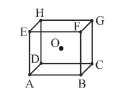A body centred cubic arrangement is shown : O is the body centre; A, B, C, D,......., H are the corners. What is the magnitude of the angle AOB ?(A) $120°$

(B) $109°28\text{'}$

(C) $104°31\text{'}$

(D) $70°32\text{'}$